# Gold inheritance

The king divided the inheritance with his three sons in the ratio of 7: 6: 4. Two of them received 286,000 gold. How much each of the sons got.

Result

a =  154000
b =  132000
c =  88000

#### Solution:

$k_{ 1 } = \dfrac{ 7 }{ 7+6+4 } = \dfrac{ 7 }{ 17 } \doteq 0.4118 \ \\ k_{ 2 } = \dfrac{ 6 }{ 7+6+4 } = \dfrac{ 6 }{ 17 } \doteq 0.3529 \ \\ k_{ 3 } = \dfrac{ 4 }{ 7+6+4 } = \dfrac{ 4 }{ 17 } \doteq 0.2353 \ \\ \ \\ t = 286000 \ \\ \ \\ z = t/(k_{ 1 }+k_{ 2 }) = 286000/(0.4118+0.3529) = 374000 \ \\ a = z \cdot \ k_{ 1 } = 374000 \cdot \ 0.4118 \doteq 154000 = 154000$
$b = z \cdot \ k_{ 2 } = 374000 \cdot \ 0.3529 \doteq 132000 = 132000$
$c = z \cdot \ k_{ 3 } = 374000 \cdot \ 0.2353 = 88000$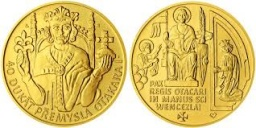Our examples were largely sent or created by pupils and students themselves. Therefore, we would be pleased if you could send us any errors you found, spelling mistakes, or rephasing the example. Thank you!

Leave us a comment of this math problem and its solution (i.e. if it is still somewhat unclear...):Be the first to comment!#### Following knowledge from mathematics are needed to solve this word math problem:

Need help calculate sum, simplify or multiply fractions? Try our fraction calculator. Most natural application of trigonometry and trigonometric functions is a calculation of the triangles. Common and less common calculations of different types of triangles offers our triangle calculator. Word trigonometry comes from Greek and literally means triangle calculation.

## Next similar math problems:

1. Red diplomasHe numbers of students with honors in 2013 and 2014 are in ratio 40:49. How big is the year-on-year percentage increase?
2. Equation with mixed fractions2 3/5 of 1430+? = 1900. How to do this problem
3. Kaufland sales-off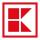As a rule the goods to the approaching end consumption in Kaufland three days before the end of the consumption to 30% off and one day before the end of the consumption to 50% discounts. Sometimes however the seller has been mistaken and the second discoun
4. Dividing moneyJanka and Silvia are to divide 1200 euros in a ratio of 19:11. How many euros does the Janka have?
5. Old laptop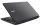The sale price of an old laptop is Php.11000. If 33 1/3% discount rate is given how much is the sale price of the laptop without the discount? Plese show your solution please. Php is Philippine peso
6. Sweets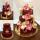3 chocolate and 7 cakes cost 85, - CZK. 2 chocolates and 6 cakes cost 86, - CZK. How much is 5 chocolates and 9 cakes? I wonder how to get the result, but only by logic without the use of a system of equations
7. Cake ingredients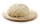The yeast cake contains milk, yeast, sugar, and flour in a ratio of 20: 4: 1: 15. What percentage of flour is in yeast?
8. Four friendsFour friends shared the money. Vasek got 1/4 of the total amount. Tonda received 1/3 of the rest of the money, Joe got a half from the second residue and Jirka left 80. How much money get together?
9. MisterMister ignacio paid Php.13693.75 for a television set that originally cost Php.15,650 . Find the rate of discount. Please show your solution please please please.
10. On a farm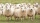On a farm, there are 90 rabbits, 700 sheep, 300 cattle and 500 pigs. What percentage of the total number of animals are rabbits?
11. Sales taxA sales tax on a Php 10,800 appliance is Php.1,620. What is the rate of sales tax?
12. Selling price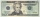Find the selling price. Cost to store: $50 Markup: 10% 13. School yearbookBianca sold Php.18,500 worth of advertisements for the school yearbook. If she is given a commision of 8%, how much did she earn for the advertisements? 14. CommissionDaniel works at a nearby electronics store. He makes a commission of 15%, percent on everything he sells. If he sells a laptop for 293.00$ how much money does Daniel make in commission?
15. Unknown number 23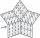Find 2/3 of unknown number, which is two thirds of the 99.
16. In the orchardIn the orchard, they planted 25 apple trees, 20 pears, 15 plums and 40 marbles. A strong late frost, however, destroyed a fifth of all new trees. Unfortunately, it was all the trees of one kind of fruit. What is the probability that the plums have died out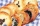Montealegre family has a catering business. In one birthday party, they charged their costumer 11,767.50 for a party of 90 persons and a service charge of 2,500.50. How much was the average cost per person? Please show your solution.